# The original eye the photo-electric effect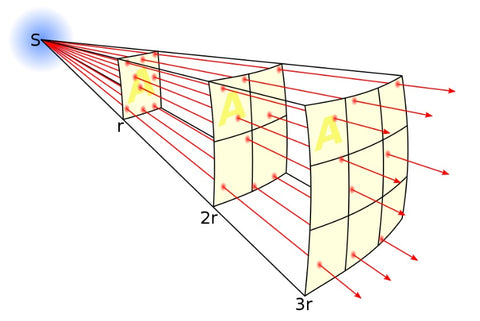Light travels in straight paths from a point line source in quanta or discrete packets. As it is written in the book of the words of Isaiah the prophet: "A voice of one calling in the wilderness, 'Prepare the way for the Lordmake straight paths for Him. The concept that light travels in straights paths, where Christ being the light, the way and the truth provides a concept in the physics of light about the behavior of light.

Light, Einstein said, is a beam of particles whose energies are related to their frequencies according to Planck's formula. When that beam is directed at a metal, the photons collide with the atoms. If a photon's frequency is sufficient to knock off an electron, the collision produces the photoelectric effect.

I am suggesting that the photon is made of electron and positron pairing so the spin states and charges are opposite and therefore the photon is massless and has a charge of zero. Newton's model of gravity can be applied where r = 1/2 the wavelength of the photon and the masses of the electron and positron are identical and the charges are opposite. Note: wavelengths of bonds in molecules are sharing of positron and electron pairs which correspond to wavelengths of light or bond length. In atoms electron and positron pair with the nucleus equal to (0,0,0) and the radius is the distance between the nucleus and the electron or positron. This concept allows the application of photon mass and charge to be inherent in a molecule when the pairing occurs and all is in the state of symmetry. In the case of a protein this is at it's isoelectric point and the balance of two proteins of opposite charge coming together to form an overall charge of zero. Zero charge being the most stable form and the lowest energy state, the preferred state for the protein to be in. This concept allows the idea of light to be applied to physical form and internal rather than external.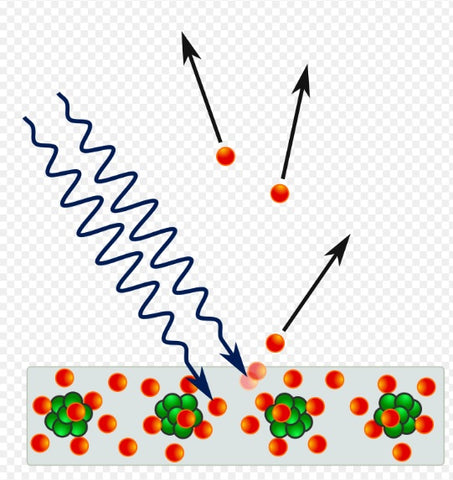This is an image of the photoelectric effect where light is seen as a wave or line and the electrons as balls. These are concepts that I do not share. The concept of duality of light being both a wave and a particle can be thought of as both at different point in time, when a photon is interacting with an electron it can be seen as a particle and when it is in free flight it can be seen as a wave. The massless and zero charge of the photon is based on its symmetry state of opposites of the electron and positron pair, which equates to the Yin yang image shown below.The yin yang concept of a photon of light (positron and electron pair) was created due to the observation of the photons being released from the photo-Fenton chemistry observed under the microscope when the components of Manuka honey were reacted together as shown in the following video.

The idea is that light (positron / electron pairs) 0 mass and 0 charge interact based on wavelengths which correspond to electron and positron pairs in atoms as long as the distance between them corresponds to the wavelength of light. So the S orbital wavelength correspond to Balmer lines or Layman lines or Paschem lines in hydrogen see below.So this image only gives you half the picture and if you look closely the line length increases but the nm number decreases so it does not make sense. If you think about the distance being between the positron and electron pairing then the wavelengths where the nucleus is 0,0,0 then the following graph can be created.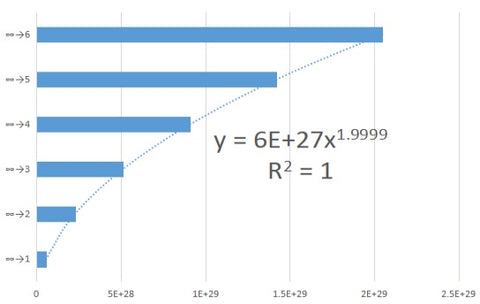The distance are in Planck lengths and correspond to the position of the orbitals of hydrogen electron positron pairs. The geometry of the electrons can be precisely determined using the Fraunhofer lines for each atom which gives the complexity of electron transitions and the photons corresponding to the wavelengths within each atom allowing distance to be determined at the atomic level. I have not gone into that detail as I considered other aspects of the concept of an electron being a point light source and the original eye.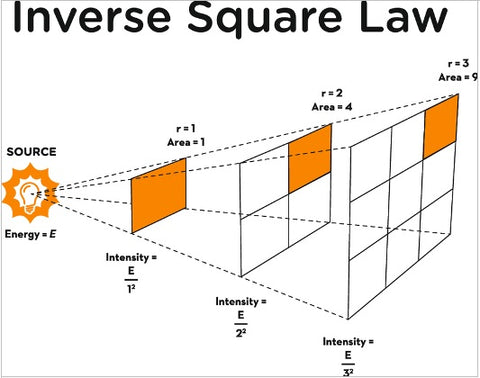Imagine each electron being its own point light source. Then use the concept that the electron is also a sphere and concave and convex lens so that the point light source gets inverted through the nucleus of 0,0,0 so that on one side it is positive and on the other side it is negative.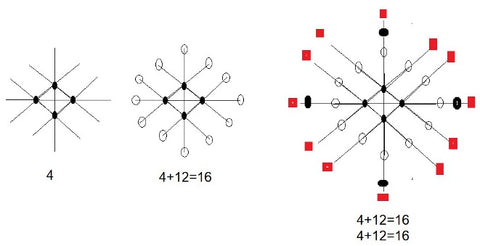So by building on this point light concept the electrons can shine through each other to create positions within the geometry of Planck lengths as outlined above where the number of S orbital electrons is 4 which enables 12 P orbital electrons to be generated. Noting the following.

The transitions correspond to molecular distances between orbitals for hydrogens single electron. If a single wavelength of light is outside of the electron transitions it cannot interact and therefore does not change the system being observed. Only the photons, wavelengths corresponding to transitions in the orbital structure can interact with the electron positron pairing and this corresponds to the spectral lines.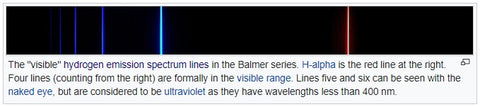In a sense the electron is a primordial eye and the sphere orbital 1S acts to reflect the inner electrons with the outer electrons in an inverse square law relationship. The Balmer lines provide the visible wavelengths of light that our retina observe from within the electron geometries within monoatomic minerals in our subconscious mind. So single atoms coordinated to our neurotransmitters can provide the original source of light within our subconscious mind. The unstable atoms releasing light at night to return back to a state of symmetry (even number of proton and neutrons) which we can observe in our dreams at night with our eyes closed.

This is my new model which includes positrons and doubles the number of electrons in each orbital. The standard model is as follows, which does not include positrons.As can be seen the current model has 2 electrons in S orbitals, 6 in p orbitals, 10 in d orbitals and 14 in f orbitals. They are paired up. So a doubling is needed because the positrons are missing to balance the charge on the -1 neutron. So my numbering is outlined below, which is built through mathematics of square law of even numbers and then built into geometry through point light source and straight line geometry and lens effects through geometric symmetry. This is able to be constructed through logical means and non measurement. The concept is that God is beyond measure where measurement is a means of disturbing symmetry therefore a asymmetry has been created through the act of measurement in order for the universe to been seen. So a correction to measured science is needed to restore the state of symmetry and when this is done the ancient knowledge is revealed. Where the Iching is based on 64 and as can be seen 64 is one of the base numbers in the electron orbitals and also there are 64 electrons in total in oxygen. So I have questioned the current model and resolved the errors introduced through measurement in the current scientific model by using logic and not measurement. Coming back to the state of symmetry and balance through the number 4.

There are 4 electrons in hydrogen. 2 x 2 = 4 and 2 + 2 = 4. This is the only combination of numbers where adding and multiplication give the same answer. The 3 and 1 concept in hydrogen of asymmetry and the 2 by 2 of symmetry (photons no mass and no charge and quantum tunneling is possible) where all forms of matter arise through the quantum tunneling of hydrogen to create other elements at a monoatomic level.

The periodic Table orbitals are n = 1: 12 = 1, n = 2: 22 = 4, n = 3: 32 = 9, n = 4: 42 = 16, n = 5: 52 = 25, n = 6: 62 = 36, n = 7: 72 = 49 and n = 8: 82 = 64The concept hold true for P and D orbitals using the point light source concept.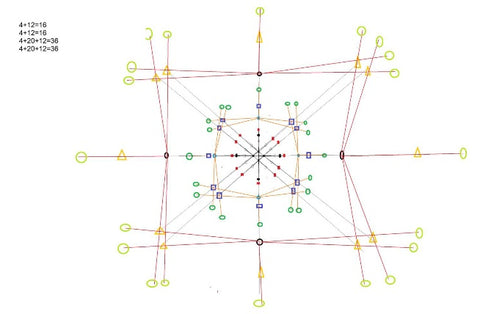D orbitals are introduced, noting that line of sight is needed as the S spherical orbital lens effect can only work with local electrons in the field, which limits the positions the electrons can occupy.F orbital electrons geometry has been introduced using the same approach creating positioning of the electrons based on direct line of sight and the electron acting as a point light source.

S orbitals have 4 electrons

P orbitals have 12 electrons

D orbitals have 20 electrons

F orbitals have 28 electrons

Orbital structure where only even numbers are stable due to symmetry an the inverse square law relationship within the periodic table of elements has been observed. This provides a logical way to appreciate the elements within the periodic table based on the inverse square law discovered by Newton.

1H n = 1: 12 = 1 4He = n = 2: 22 = 4

4 + 12 =16 n = 4: 42 = 16

4 + 12 = 16 n = 4: 42 = 16

4 + 20 + 12 = 36 n = 6: 62 = 36

4 + 20 + 12 = 36 n = 6: 62 = 36

4+ 28 + 20 + 12 = 64 n = 8: 82 = 64

4+ 28 + 20 + 12 = 64 n = 8: 82 = 64

The symmetry in the orbital layers provides the concept of stability through symmetry of opposites and Yin yang concept. Inverse square law or Newton's law. Only the even numbers are stable. Why? Well the duality of opposites creates stability and therefore we see this play out in the atomic world where even numbered atoms are more abundant than odd numbered elements. See below.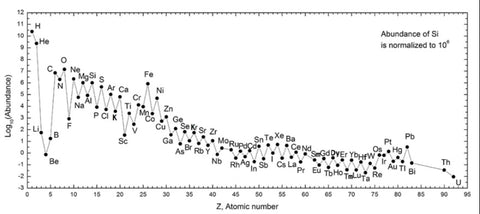So uneven numbers create unstable elements as pairing cannot occur between electron and positrons and quarks in the neutrons and protons. With an uneven element being less stable due to the asymmetry within in its structure. Unstable elements being radioactive and a source of inner atomic energy that biology uses.

I believe it is this instability that is responsible for life and not the stable form of elements that biology has focused on due to the fact that the speed of transmutation and energy release is greater than our conscious awareness allows us to see, therefore we are consciously unaware of the physics happening within us which needs no enzymes to modulate its rate of reaction but is tuned by atomic geometries and atomic half lives. I do not think we have any semblance of control in this state of higher elevation but feel a blissful state of euphoria as energy is released within and at an atomic level that is beyond our biological cognition and permeates as an essence of pure divinity within. A state of radiance and pure Love. This self awareness of the light within is an elevated consciousness. Seen by the external person as mania because more energy is being released than can be comprehended and in this state less sleep is needed and energy levels are elevated. Again I see this as a connection to the physics of the human being and natural and not some pathology or disease state. In this state learning is happening rapidly and transformation can occur.